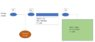# Ideal Gas Law problem

Catstranaughts
Homework Statement:
Find Volume of gasline: I have known pressure chamber (vacuum) that P= 1e-6 Torr. Volume is 101L. T = 25C. I can easily solve for n. But now I have a gasline with an unknown volume which I'm trying to calculate. I know the pressure of the gasline. but I also don't know the number of molecules in the gas line.
Relevant Equations:
PV =nRT
Please refer to diagram. V1 is open initially then V2 is open for 5 minutes for pressure to equalize. V1 and V2 are then shut. V3 is opened. What is Vol 2 ? P(final)*V(final) = n(final)* R*T => (Vol1 + Vol2) = n(final)*R*25C/ 0.070 Torr where n(final) = n(Vol1) + n(Vol2)

If I shut V3, I can find the n(Vol 1), but i still don's know n(gasline) so I can't find Vol 2. Is there enough information given to solve this problem ?

#### Attachments

•ideal gas law.JPG
19 KB · Views: 152

Homework Helper
Gold Member
2022 Award
If I understand the problem statement, you are given all the conditions prior to opening V3 except Vol2, and no final conditions. If so, it is clear that there is insufficient information. You can make Vol2 whatever you like and no facts are violated.
Please check that no final conditions are given.

Homework Helper
Gold Member
Just a note on your formula: PV = nRT. Make sure your temperature is absolute (either Kelvin or Rankine). Also, your units for volume and pressure should be consistent with the units for R.

Catstranaughts
If I understand the problem statement, you are given all the conditions prior to opening V3 except Vol2, and no final conditions. If so, it is clear that there is insufficient information. You can make Vol2 whatever you like and no facts are violated.
Please check that no final conditions are given.
Thanks haruspex. This is in a lab. There is a mass flow controller in the gasline. But this leaves the volume measurement dependent upon the accuracy of the MFC and gas correction factor associated with it. Is there other information I can gather to help solve this ?

Just a note on your formula: PV = nRT. Make sure your temperature is absolute (either Kelvin or Rankine). Also, your units for volume and pressure should be consistent with the units for R.
Thanks Dave, I'm converting to K and atm (and using liters)

Homework Helper
Gold Member
2022 Award
This is in a lab.
So what measurements were taken after opening V3?
There is a mass flow controller in the gasline.
You'd need to explain to me what that does.

Catstranaughts
So what measurements were taken after opening V3?
Pressure in the system by a capacitance manometer. Pressure before V3 is open: P(gasline) = 760 Torr. P(chamber = 1e-6 Torr). Pressure after V3 open: P Gasline = Pressure Chamber = 70mTorr.
You'd need to explain to me what that does.
For the test, the Mass Flow Controller was programmed to max value to fully open needle valve. There is also a V4 that is opened (that bypasses the MFC)